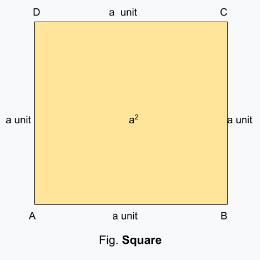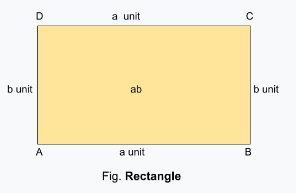Algebraic Identity (a - b)²

## Objective

To verify the algebraic identity (a-b)² = a² - 2ab + b².

## Algebraic Identity

• An algebraic identity is an equality, which is true for all values of the variables in the equality.
• The algebraic equations which are valid for all values of variables in them are called algebraic identities.
• In this way, algebraic identities are used in the computation of algebraic expressions and solving different polynomials
• Algebraic identities are also used for the factorization of polynomials.

## SquareA square is a regular quadrilateral, which means that it has four equal sides and four equal angles of 90 degrees each.

## Area of a Square

• The product of the length of each side itself.
• Formula: Area = side²

## RectangleRectangle is a quadrilateral with four right angles and opposite sides are equal. The length of a rectangle is the size of the longer side, whereas the breadth is the size of the shorter side

## Area of a Rectangle

• The product of its length and breadth
• Formula: Area = length x breadth